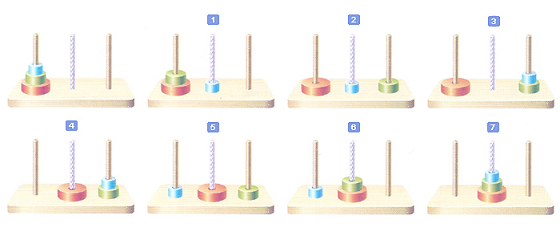Probability

# Linear Recurrence Relations - Problem SolvingA quiz contest has the following rules:

1. There are 10 stages to pass through.
2. For passing through the first stage, 50 points are given.
3. For passing through the $n^{\text{th}}$ stage, additional $(5n + 20)$ points are given, where $n=2, 3, \ldots, 9, 10.$

If someone passes through all 10 stages, what is the final score?The above is an illustration of the 7 steps in which a tower of three stones stacked onto a rod in ascending order of size is moved to the target rod in the middle, using a vacant rod on the right. Each of the seven moves obeys the following rules:

1) Only one disk at the top of a stack can be moved at a time.
2) No disk can be placed on top of a smaller disk.

What is the minimum number of steps required to move a tower of 7 disks?A tower is built with cards, as illustrated above. Only 2 cards are needed for 1 floor, and 7 cards are needed for 2 floors (as shown above), and so on. What is the number of cards needed for a 10-floor tower?A beach umbrella is divided into 6 sectors, where one has a larger area than all the other five with the same area. If the 6 sectors are to be painted with 3 colors and adjacent sectors must have different colors, how many different ways are there?

Details and assumptions

• There can be colors not used.There are 10 bacteria in a flask. Every hour, 3 bacteria die and the remaining ones are each divided into 2. After 1 day, how many bacteria will live there?

Assume that the flask is large enough to contain any number of bacteria.

×